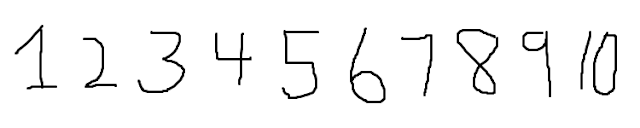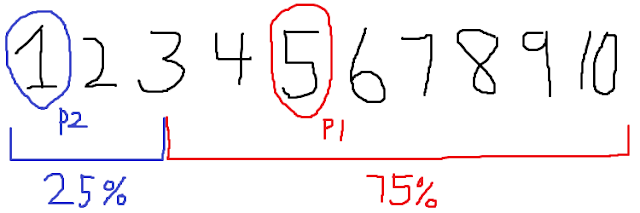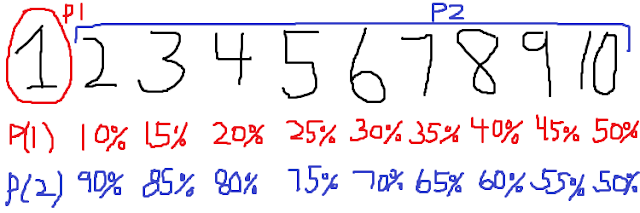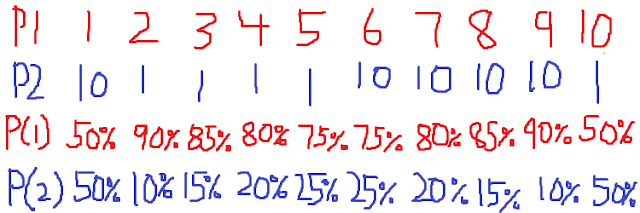## June 04, 2014

### Between One and Ten

Here's some background information: I was a campus tour guide at Brown University, which means that for one hour every week, I had to give a tour to prospective students and parents who are interested in the university. There were quite a few of us and we are all assigned individual time slots. On my slot, (Monday, 3PM) there were three other tour guides for a total of four.

Now here's the scenario: there were three tour guides one day (one was sick) and about four prospective students. Clearly, only a single tour guide was necessary. But how do we choose which of us three has to give the tour in a fair and diplomatic way? Do we play rock-paper-scissors? But that could take ages.

Here's the method: each of the tour guides picks a number from one to ten (inclusive). Then a prospective student does the same. Whoever is closest to the student's number has to give the tour. The others picked three and five, while I picked four. The number was five. I did not have to give the tour.

Here's the problem: On the walk back to our student center, the other tour guide who picked three said to me that my method was incredibly fair and she was very surprised at how quickly I had come up with it. This led me to think--is it really all that fair? Is there a way to pick a number so as to minimize my chances of giving the tour? I guess it's time for some economics.One through ten
As you can see from above, there are exactly ten choices that we could have picked. To start off, we assume that each number is equally likely to be picked with a probability of 10%. We also set that no two players may pick the same number. Therefore the game is also a consecutive one--players take turns picking numbers, with only the prospective student picking any number he/she wishes. Again, to reiterate the rules, the player closest to the prospective's number must give the tour. So we must be as far from that number as possible to minimize our chances of giving the tour. (Because college students are lazy).

In the above scenario, there were three players in the game. Let's first simplify the problem and pretend that there were only two tour guides--player 1 (P1), player 2 (P2), and the prospective student (PS).

How does the game play out?
1. PS chooses a number independently.
2. P1 chooses a number first.
3. P2 chooses last.
Let's say P1 chooses the number 5 arbitrarily. What is the best response for P2? Intuitively we can see that the number 1 is the best choice to minimize the chances of giving the tour.If P1 = 5, then P2 should play 1
What happens if the PS is 3? Then a fair coin would be used to decide who gives the tour. Therefore, as the above diagram depicts, there is a 75% chance that P1 gives the tour, whereas P2 only has a 25% chance.

Now let's say P1 knew all that, so he decides to choose 1 before P2 has the chance to. What is the best response for P2 now? We can easily calculate the probabilities for every other choice as such:Probabilities of both players if P1 first picks 1
We can see from the above chart that if P1 first picks 1, then P2 minimizes his/her chances of giving a tour by picking 10. Knowing these probabilities, how would the rational person behave? We could derive a large 10 by 10 payoff matrix listing all the possibilities, but that would be too much. Instead, we can intuitively see that, assuming both players are rational (i.e. economics majors), that the Nash equilibrium is (1, 10) or (10, 1) with the probabilities of either player giving a tour at 50%. Not a very interesting conclusion.

But what if P1 is not rational? What if P1 isn't an economics major? What is the best response of P2 given any choice that P1 can make?P1's choice, the best response of P2, and the respective probabilities
As you can see from the above chart, given any choice made by P1, the best response by P2 is either 1 or 10. But how is that determined? It's easy to see that P2 should pick the furthest number on the side with the least amount of numbers on it. So for example, if P1 picked 6, there are 4 numbers to its right, and 5 numbers to the left. The furthest number on the right is 10, so therefore P2 should pick 10 for the minimum probability of 25%. This rule stands except for the case of 1 and 10, in which case there is only one side to pick from.

What have we learned so far? What are the valid conclusions?
• If you are P1:
• Is P2 rational?
• Play 1 or 10
• Is P2 irrational?
• Cannot tell what P2 will pick, but still pick 1 or 10 to minimize probability
• If you are P2:
• Is P1 rational?
• Play 1 or 10, whichever P1 did not pick
• Is P2 irrational?
• Play the furthest number from P1's choice on the side with the least amount of numbers